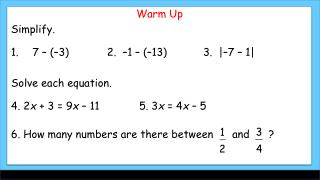# Warm Up Simplify. 7 – (–3 ) 2 . – 1 – (–13 ) 3. | – 7 – 1| Solve each equation . - PowerPoint PPT PresentationDownload PresentationWarm Up Simplify. 7 – (–3 ) 2 . – 1 – (–13 ) 3. | – 7 – 1| Solve each equation .

Download Presentation## Warm Up Simplify. 7 – (–3 ) 2 . – 1 – (–13 ) 3. | – 7 – 1| Solve each equation .

- - - - - - - - - - - - - - - - - - - - - - - - - - - E N D - - - - - - - - - - - - - - - - - - - - - - - - - - -
##### Presentation Transcript

1. Warm Up Simplify. 7 – (–3) 2. –1 – (–13) 3. |–7 – 1| Solve each equation. 4. 2x + 3 = 9x – 11 5. 3x = 4x – 5 6. How many numbers are there between and ?

2. Warm Up Simplify. 7 – (–3) 2. –1 – (–13) 3. |–7 – 1| Solve each equation. 4. 2x + 3 = 9x – 11 5. 3x = 4x – 5 6. How many numbers are there between and ? 10 12 8 x = 2 x = 5 Infinitely many

3. Vocabulary coordinate midpoint distance bisect length segment bisector construction between congruent segments

4. A ruler can be used to measure the distance between two points. A point corresponds to one and only one number on a ruler. The number is called a coordinate. The following postulate summarizes this concept.

5. A B AB = |a– b| or |b - a| a b The distance between any two points is always positive. Therefore, we can say that the distance between the points is the absolute value of the difference of the coordinates. If the coordinates of points A and B are a and b, then the distance between A and B is |a – b| or |b – a|. The distance between A and B is also called the length of AB, or AB.

6. Congruent segments are segments that have the same length. Use compass only – no ruler – to create congruent segments Create congruent segments on your notes (lightly show tick mark).

7. Key Concept – Be careful … PQ represents a number (or distance) while represents a geometric figure (line segment). Be sure to use equality for numbers (PQ = PQ) while we use congruence for figures

8. In order for you to say that a point B is between two points A and C, all three points must lie on the same line (which is to say they are collinear) and AB + BC = AC.

9. M is between N and O. Find NO. The key to solving this is the segment addition postulate which says than NM + MO = NO

10. The midpointM of AB is the point that bisects, or divides, the segment into two congruent segments. If M is the midpoint of AB, then AM = MB. So if AB = 6, then AM = 3 and MB = 3. Bisect a segment with a compass …

11. Lesson Quiz 1.Mis between N and O. MO = 15, and MN = 7.6. Find NO. Sis the midpoint of, TS = 4x – 7, andSV= 5x – 15. Find TS, SV, and TV. LH bisects GK at M. GM =2x + 6, and GK = 24.Findx.

12. Lesson Quiz 1.Mis between N and O. MO = 15, and MN = 7.6. Find NO. NO = 22.6 Sis the midpoint of, TS = 4x – 7, andSV= 5x – 15. Find TS, SV, and TV. LH bisects GK at M. GM =2x + 6, and GK = 24.Findx.

13. Lesson Quiz 1.Mis between N and O. MO = 15, and MN = 7.6. Find NO. Sis the midpoint of, TS = 4x – 7, andSV= 5x – 15. Find TS, SV, and TV. TS = 25, SV = 25 and TV = 50 LH bisects GK at M. GM =2x + 6, and GK = 24.Findx.

14. Lesson Quiz 1.Mis between N and O. MO = 15, and MN = 7.6. Find NO. Sis the midpoint of, TS = 4x – 7, andSV= 5x – 15. Find TS, SV, and TV. LH bisects GK at M. GM =2x + 6, and GK = 24.Findx. x = 3

15. Assignment today is page 17: 12-15, 20-29, 35-41 and 48-49. Remember that homework help is always available at http://www.thinkcentral.com/index.htm Today’s keyword is “MG7 1-2”.